# MIRACLE data analysis tools

## 7)Elementary Current Method (ECM) for combining multi-satellite FAC data and ground magnetometer data to obtain distributions of true ionospheric currents:

### - Short description of the analysis method:

The Elementary Current Method (ECM) combines spatial ground magnetic field measurements from a magnetometer network (like IMAGE) with field-aligned current (FAC) estimates from a fleet of satellites (like CLUSTER II), mapped down to the ionosphere, to calculate 2D distributions of the true (not equivalent) ionospheric currents.
The basic idea is that the ground magnetic measurements give the curl of the true current system, while the FAC give its divergence. This information is combined to the wanted true current system by using spherical elementary current systems that represent "point sources" (Green's functions) of the curl and divergence operator on the sphere.
If additionally the ionospheric electric field is measured on the analysis area by a coherent scatter radar (like STARE), also the Hall and Pedersen conductance distributions can be calculated.

For more details: see Literature (below)

### - Input data requirements:

Spatial input data of the ground magnetic field, and of the field-aligned currents (FACs) as measured by the satellites, mapped down to the ionosphere.
To obtain 2D FAC distributions from the satellites, it is of advantage if the ionospheric situation to be studied can be assumed stationary for the time that either:
• the ionospheric feature moves over the satellites' footpoints, or
• the satellites' footpoints move over the analysis region selected, or
• a combination of the above.
Then, FAC data of different timesteps can be reduced to a reference timestep, with respect to the velocity of the ionospheric feature and/or of the satellites' footpoints, to obtain a more complete 2D FAC distribution.

### - Output quantities:

Spatial distributions of true ionospheric currents. If 2D electric field data is available on the modeling region, spatial distributions of conductances are an additional output.

### - Literature:

• Amm, O., The elementary current method for calculating ionospheric current systems from multi-satellite and ground magnetometer data, J. Geophys. Res., 106, 24843, 2001.
• Amm, O., K. Kauristie, T.I. Pulkkinen, M.J. Engebretson, R.A. Greenwald, H. Lühr, and T. Moretto, Combining multi-point spacecraft and two-dimensional ground-based observations: Theory and example of an IMF BY-related cusp current system, Proc. Cluster II workshop, ESA SP-449, 327, 1999.
• Amm, O., Ionospheric elementary current systems in spherical coordinates and their application, J. Geomagn. Geoelectr., 49, 947, 1997.

### - Example plots for a modeled case of a dark plasma vortex:

To illustrate the method, we use a model of a dark plasma vortex that moves eastward over the MIRACLE field of view with a velocity of 4 km/s. We assume that the vortex is stationary for the 2 minutes that it needs to pass over the central MIRACLE field of view. The model of the plasma vortex comprises the main results of a real event study of such an ionospheric feature that was recently conducted by Kosch et al. (JGR 105, 24889, 2000).

This is the input data: FAC distribution that CLUSTER II would observe during the 2 minutes when the vortex passes eastward over the MIRACLE array. The FAC data of the different timesteps is reduced to a central reference timestep with respect to the vortex's velocity (upper panel). The footpoints and conjugate magnetic field lines of the CLUSTER II satellites at the reference timestep are shown in the lower panel, for a typical pass over the central MIRACLE field of view.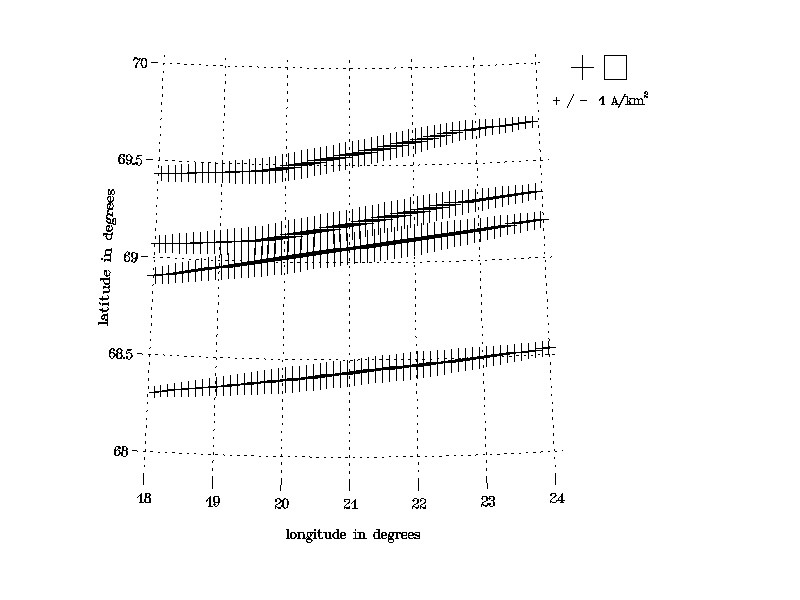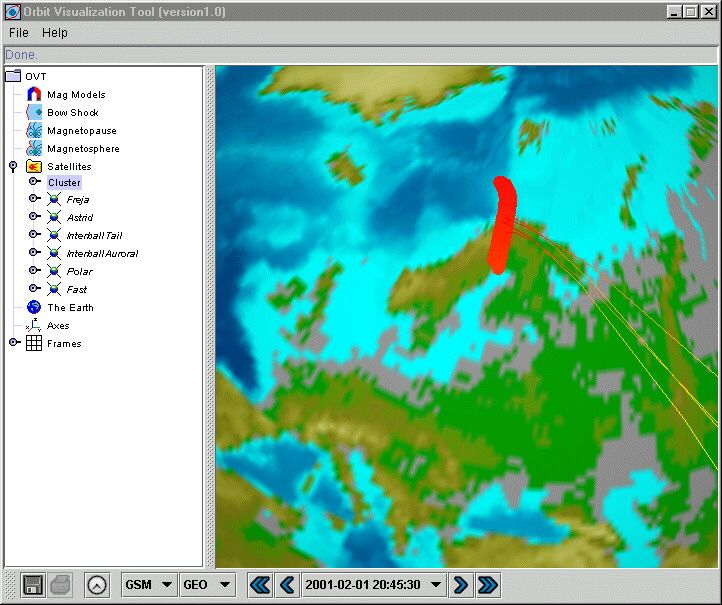Ground magnetic field signature (rotated clockwise by 90 degrees) that the IMAGE network would observe at the reference timestep (numbers: vertical downward component):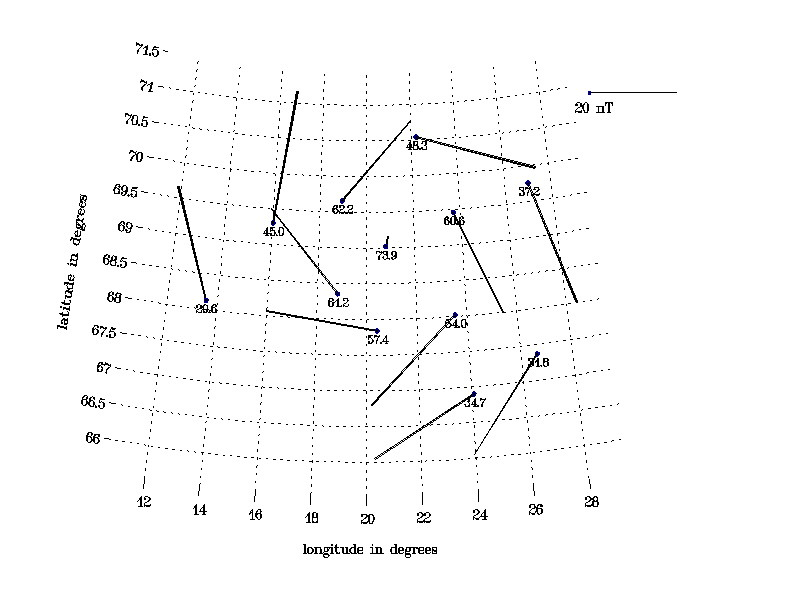Output:True (not equivalent) ionospheric currents obtained by the Elementary Current Method (ECM):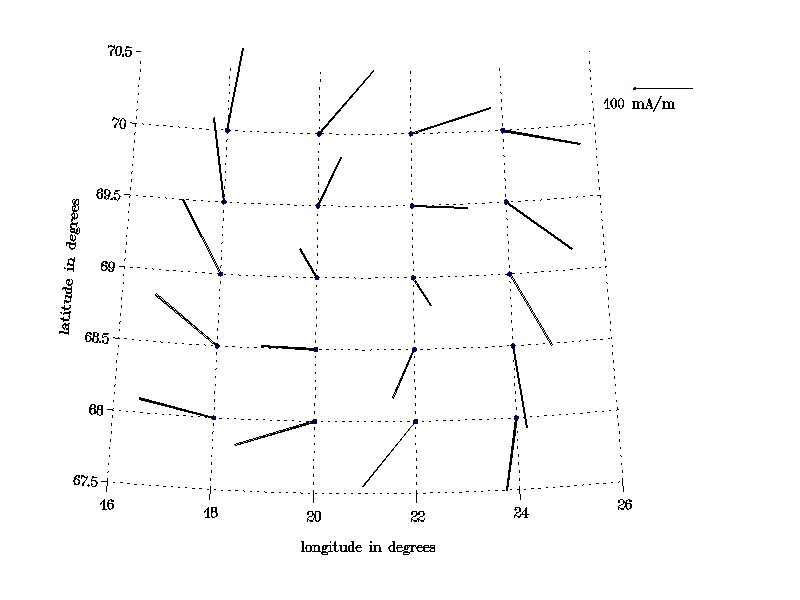- Optional, additional input: Electric field distribution measured by the STARE coherent scatter radar:-> Additional output: Hall conductance distribution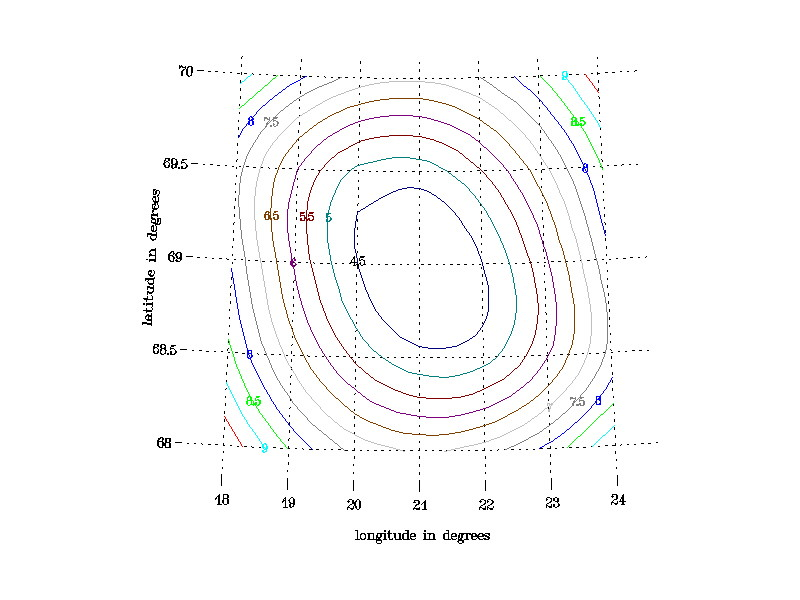and Pedersen conductance distribution: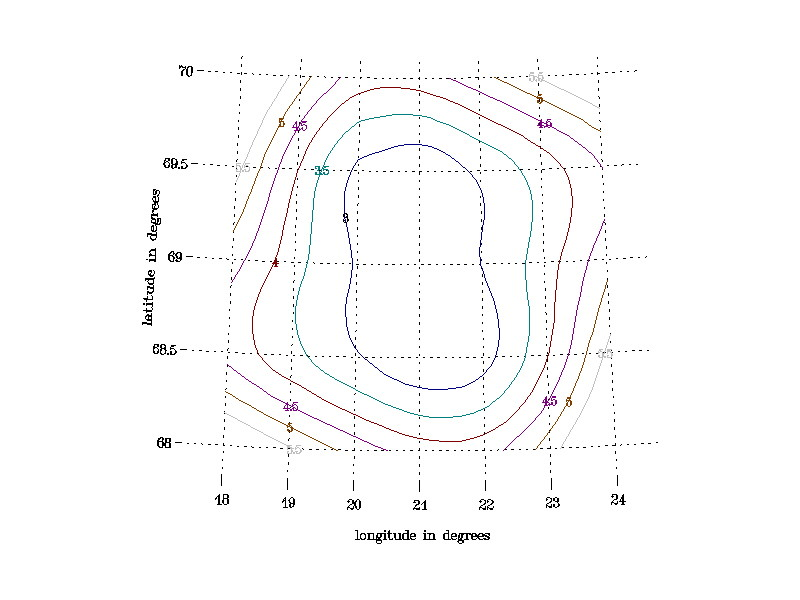For further questions and proposals of events to be analysed, contact Olaf Amm.

Maintained by: Olaf Amm
Latest update: 18.03.2004